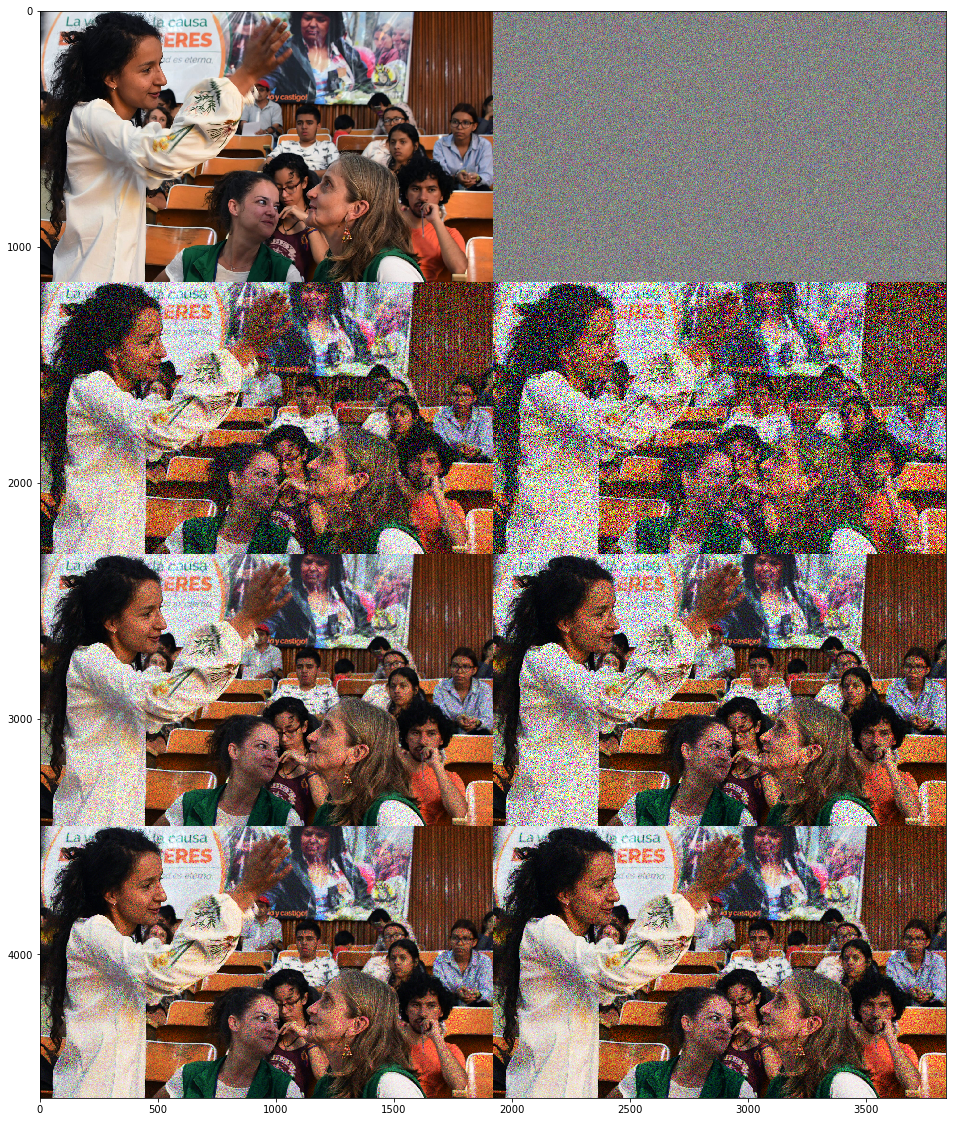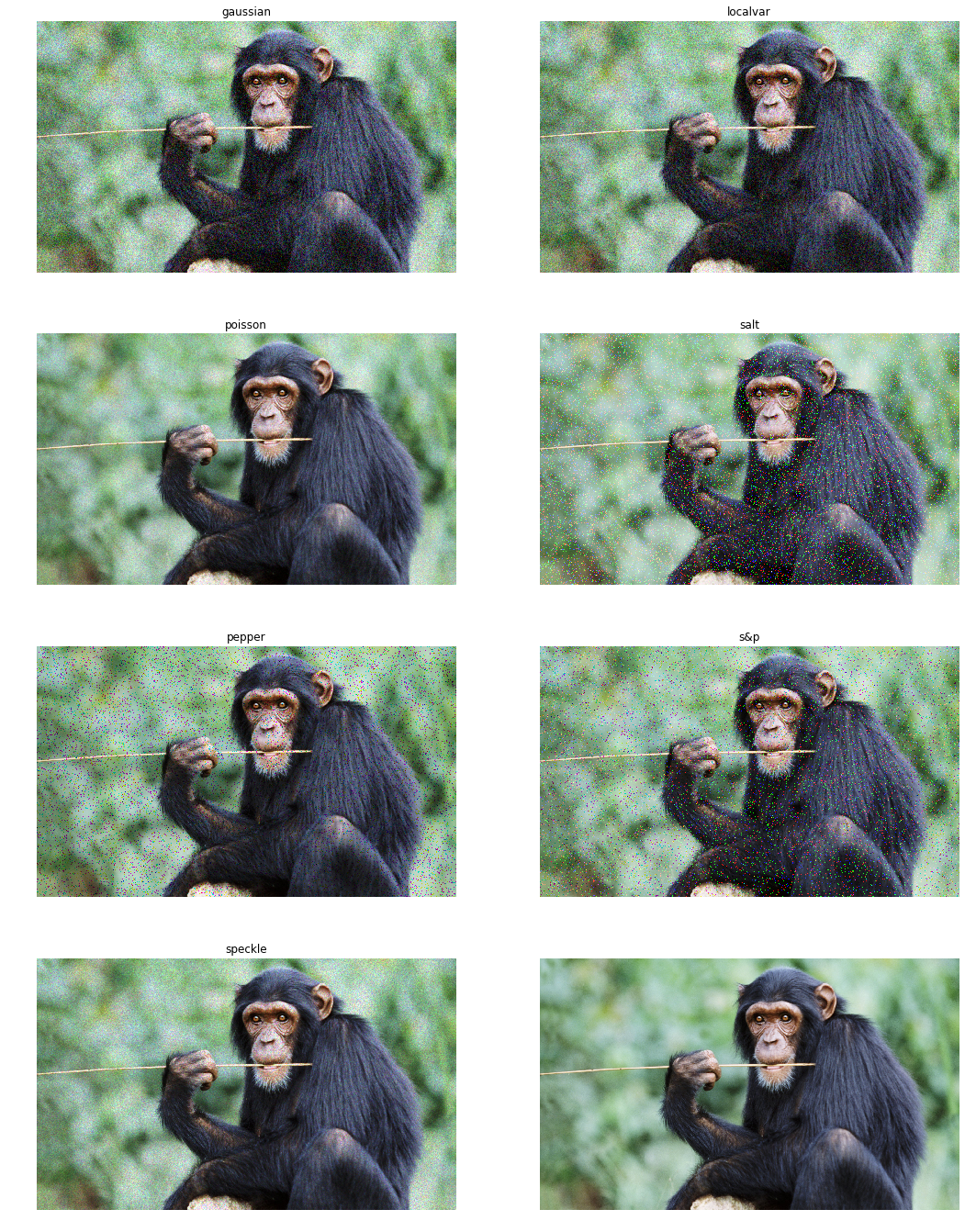Instantly share code, notes, and snippets.

Last active July 28, 2023 03:55
Star You must be signed in to star a gist
Python code to add random Gaussian noise on images
This file contains bidirectional Unicode text that may be interpreted or compiled differently than what appears below. To review, open the file in an editor that reveals hidden Unicode characters. Learn more about bidirectional Unicode characters
 import cv2 def add_gaussian_noise(X_imgs): gaussian_noise_imgs = [] row, col, _ = X_imgs.shape # Gaussian distribution parameters mean = 0 var = 0.1 sigma = var ** 0.5 for X_img in X_imgs: gaussian = np.random.random((row, col, 1)).astype(np.float32) gaussian = np.concatenate((gaussian, gaussian, gaussian), axis = 2) gaussian_img = cv2.addWeighted(X_img, 0.75, 0.25 * gaussian, 0.25, 0) gaussian_noise_imgs.append(gaussian_img) gaussian_noise_imgs = np.array(gaussian_noise_imgs, dtype = np.float32) return gaussian_noise_imgs gaussian_noise_imgs = add_gaussian_noise(X_imgs)

### liruoteng commented Jan 24, 2019

where did you use the defined 'var' and 'sigma'?

### b-safwat commented Mar 8, 2019 • edited

it shall be:
` gaussian = np.random.normal(mean,sigma,(row,col, 1))`
` gaussian = np.random.random((row, col, 1)).astype(np.float32)`

### b-safwat commented Mar 8, 2019 • edited

Also Note that this is not adding gaussian noise, it adds a transparent layer to make the image darker (as if it is changing the lighting)

Adding gaussian noise shall looks like so:

``````import numpy as np
import cv2
mean = 0
var = 10
sigma = var ** 0.5
gaussian = np.random.normal(mean, sigma, (224, 224)) #  np.zeros((224, 224), np.float32)

noisy_image = np.zeros(img.shape, np.float32)

if len(img.shape) == 2:
noisy_image = img + gaussian
else:
noisy_image[:, :, 0] = img[:, :, 0] + gaussian
noisy_image[:, :, 1] = img[:, :, 1] + gaussian
noisy_image[:, :, 2] = img[:, :, 2] + gaussian

cv2.normalize(noisy_image, noisy_image, 0, 255, cv2.NORM_MINMAX, dtype=-1)
noisy_image = noisy_image.astype(np.uint8)

cv2.imshow("img", img)
cv2.imshow("gaussian", gaussian)
cv2.imshow("noisy", noisy_image)

cv2.waitKey(0)

``````

### xvdp commented May 14, 2019

3 examples,

• gaussian noise multiplied then added over image: noise increases with image value
• image folded over and gaussian noise multipled and added to it: peak noise affects mid values, white and black receiving little noise
in every case i blend in 0.2 and 0.4 of the image
```import numpy as np
import cv2
import matplotlib.pyplot as plt

noise =  np.random.normal(loc=0, scale=1, size=img.shape)

# noise overlaid over image
noisy = np.clip((img + noise*0.2),0,1)
noisy2 = np.clip((img + noise*0.4),0,1)

# noise multiplied by image:
# whites can go to black but blacks cannot go to white
noisy2mul = np.clip((img*(1 + noise*0.2)),0,1)
noisy4mul = np.clip((img*(1 + noise*0.4)),0,1)

noisy2mul = np.clip((img*(1 + noise*0.2)),0,1)
noisy4mul = np.clip((img*(1 + noise*0.4)),0,1)

# noise multiplied by bottom and top half images,
# whites stay white blacks black, noise is added to center
img2 = img*2
n2 = np.clip(np.where(img2 <= 1, (img2*(1 + noise*0.2)), (1-img2+1)*(1 + noise*0.2)*-1 + 2)/2, 0,1)
n4 = np.clip(np.where(img2 <= 1, (img2*(1 + noise*0.4)), (1-img2+1)*(1 + noise*0.4)*-1 + 2)/2, 0,1)

# norm noise for viz only
noise2 = (noise - noise.min())/(noise.max()-noise.min())
plt.figure(figsize=(20,20))
plt.imshow(np.vstack((np.hstack((img, noise2)),
np.hstack((noisy, noisy2)),
np.hstack((noisy2mul, noisy4mul)),
np.hstack((n2, n4)))))
plt.show()
plt.hist(noise.ravel(), bins=100)
plt.show()```### xvdp commented Jun 2, 2019 • edited

skimage has a few nice noise functions easy to compare, i like poisson, looks closest to film grain

```import skimage
import matplotlib.pyplot as plt
img_path="https://i.guim.co.uk/img/media/4ddba561156645952502f7241bd1a64abd0e48a3/0_1251_3712_2225/master/3712.jpg?width=1920&quality=85&auto=format&fit=max&s=1280341b186f8352416517fc997cd7da"

def plotnoise(img, mode, r, c, i):
plt.subplot(r,c,i)
if mode is not None:
gimg = skimage.util.random_noise(img, mode=mode)
plt.imshow(gimg)
else:
plt.imshow(img)
plt.title(mode)
plt.axis("off")

plt.figure(figsize=(18,24))
r=4
c=2
plotnoise(img, "gaussian", r,c,1)
plotnoise(img, "localvar", r,c,2)
plotnoise(img, "poisson", r,c,3)
plotnoise(img, "salt", r,c,4)
plotnoise(img, "pepper", r,c,5)
plotnoise(img, "s&p", r,c,6)
plotnoise(img, "speckle", r,c,7)
plotnoise(img, None, r,c,8)
plt.show()```### PallawiSinghal commented Oct 12, 2019

skimage has a few nice noise functions easy to compare, i like poisson, looks closest to film grain

```import skimage
import matplotlib.pyplot as plt
img_path="https://i.guim.co.uk/img/media/4ddba561156645952502f7241bd1a64abd0e48a3/0_1251_3712_2225/master/3712.jpg?width=1920&quality=85&auto=format&fit=max&s=1280341b186f8352416517fc997cd7da"

def plotnoise(img, mode, r, c, i):
plt.subplot(r,c,i)
if mode is not None:
gimg = skimage.util.random_noise(img, mode=mode)
plt.imshow(gimg)
else:
plt.imshow(img)
plt.title(mode)
plt.axis("off")

plt.figure(figsize=(18,24))
r=4
c=2
plotnoise(img, "gaussian", r,c,1)
plotnoise(img, "localvar", r,c,2)
plotnoise(img, "poisson", r,c,3)
plotnoise(img, "salt", r,c,4)
plotnoise(img, "pepper", r,c,5)
plotnoise(img, "s&p", r,c,6)
plotnoise(img, "speckle", r,c,7)
plotnoise(img, None, r,c,8)
plt.show()```awesome

### quantshah commented Jan 28, 2020

This is so cool. Thank you so much.

### RehamMuhammad commented Apr 25, 2020

3 examples,

• gaussian noise multiplied then added over image: noise increases with image value
• image folded over and gaussian noise multipled and added to it: peak noise affects mid values, white and black receiving little noise
in every case i blend in 0.2 and 0.4 of the image
```import numpy as np
import cv2
import matplotlib.pyplot as plt

noise =  np.random.normal(loc=0, scale=1, size=img.shape)

# noise overlaid over image
noisy = np.clip((img + noise*0.2),0,1)
noisy2 = np.clip((img + noise*0.4),0,1)

# noise multiplied by image:
# whites can go to black but blacks cannot go to white
noisy2mul = np.clip((img*(1 + noise*0.2)),0,1)
noisy4mul = np.clip((img*(1 + noise*0.4)),0,1)

noisy2mul = np.clip((img*(1 + noise*0.2)),0,1)
noisy4mul = np.clip((img*(1 + noise*0.4)),0,1)

# noise multiplied by bottom and top half images,
# whites stay white blacks black, noise is added to center
img2 = img*2
n2 = np.clip(np.where(img2 <= 1, (img2*(1 + noise*0.2)), (1-img2+1)*(1 + noise*0.2)*-1 + 2)/2, 0,1)
n4 = np.clip(np.where(img2 <= 1, (img2*(1 + noise*0.4)), (1-img2+1)*(1 + noise*0.4)*-1 + 2)/2, 0,1)

# norm noise for viz only
noise2 = (noise - noise.min())/(noise.max()-noise.min())
plt.figure(figsize=(20,20))
plt.imshow(np.vstack((np.hstack((img, noise2)),
np.hstack((noisy, noisy2)),
np.hstack((noisy2mul, noisy4mul)),
np.hstack((n2, n4)))))
plt.show()
plt.hist(noise.ravel(), bins=100)
plt.show()```Thank you so much that was so helpful

### Guardian99 commented May 29, 2020

3 examples,

• gaussian noise multiplied then added over image: noise increases with image value
• image folded over and gaussian noise multipled and added to it: peak noise affects mid values, white and black receiving little noise
in every case i blend in 0.2 and 0.4 of the image
```import numpy as np
import cv2
import matplotlib.pyplot as plt

noise =  np.random.normal(loc=0, scale=1, size=img.shape)

# noise overlaid over image
noisy = np.clip((img + noise*0.2),0,1)
noisy2 = np.clip((img + noise*0.4),0,1)

# noise multiplied by image:
# whites can go to black but blacks cannot go to white
noisy2mul = np.clip((img*(1 + noise*0.2)),0,1)
noisy4mul = np.clip((img*(1 + noise*0.4)),0,1)

noisy2mul = np.clip((img*(1 + noise*0.2)),0,1)
noisy4mul = np.clip((img*(1 + noise*0.4)),0,1)

# noise multiplied by bottom and top half images,
# whites stay white blacks black, noise is added to center
img2 = img*2
n2 = np.clip(np.where(img2 <= 1, (img2*(1 + noise*0.2)), (1-img2+1)*(1 + noise*0.2)*-1 + 2)/2, 0,1)
n4 = np.clip(np.where(img2 <= 1, (img2*(1 + noise*0.4)), (1-img2+1)*(1 + noise*0.4)*-1 + 2)/2, 0,1)

# norm noise for viz only
noise2 = (noise - noise.min())/(noise.max()-noise.min())
plt.figure(figsize=(20,20))
plt.imshow(np.vstack((np.hstack((img, noise2)),
np.hstack((noisy, noisy2)),
np.hstack((noisy2mul, noisy4mul)),
np.hstack((n2, n4)))))
plt.show()
plt.hist(noise.ravel(), bins=100)
plt.show()```Is there a way to add noise to the bottom half of the image?

### IrshadAhmedHanif commented Mar 20, 2021

I have an issue in line 5..
'str' object has no attribute 'shape'
how can i solve this issue?

### rajiv1990 commented Mar 22, 2021

I have an issue in line 5..
'str' object has no attribute 'shape'

Appears like the parameter you have sent is a string.
You can try reading an image file by using cv2.imread() and send that to the function

### santykish23 commented Mar 1, 2022

Depends what your goal is: 3 examples,

``````* gaussian noise added over image: noise is spread throughout

* gaussian noise multiplied then added over image: noise increases with image value

* image folded over and gaussian noise multipled and added to it: peak noise affects mid values, white and black receiving little noise
in every case i blend in 0.2 and 0.4 of the image
``````
```import numpy as np
import cv2
import matplotlib.pyplot as plt

noise =  np.random.normal(loc=0, scale=1, size=img.shape)

# noise overlaid over image
noisy = np.clip((img + noise*0.2),0,1)
noisy2 = np.clip((img + noise*0.4),0,1)

# noise multiplied by image:
# whites can go to black but blacks cannot go to white
noisy2mul = np.clip((img*(1 + noise*0.2)),0,1)
noisy4mul = np.clip((img*(1 + noise*0.4)),0,1)

noisy2mul = np.clip((img*(1 + noise*0.2)),0,1)
noisy4mul = np.clip((img*(1 + noise*0.4)),0,1)

# noise multiplied by bottom and top half images,
# whites stay white blacks black, noise is added to center
img2 = img*2
n2 = np.clip(np.where(img2 <= 1, (img2*(1 + noise*0.2)), (1-img2+1)*(1 + noise*0.2)*-1 + 2)/2, 0,1)
n4 = np.clip(np.where(img2 <= 1, (img2*(1 + noise*0.4)), (1-img2+1)*(1 + noise*0.4)*-1 + 2)/2, 0,1)

# norm noise for viz only
noise2 = (noise - noise.min())/(noise.max()-noise.min())
plt.figure(figsize=(20,20))
plt.imshow(np.vstack((np.hstack((img, noise2)),
np.hstack((noisy, noisy2)),
np.hstack((noisy2mul, noisy4mul)),
np.hstack((n2, n4)))))
plt.show()
plt.hist(noise.ravel(), bins=100)
plt.show()```img = cv2.imread(img_path)[...,::-1]/255.0 in this line what why [...,-1]/255.0] is used? please explain this !!!

### santykish23 commented Mar 1, 2022

I have an issue in line 5.. 'str' object has no attribute 'shape' how can i solve this issue?

bro, could you explain this line, img = cv2.imread(img_path)[...,::-1]/255.0 in this line what why [...,-1]/255.0] is used?

### xvdp commented Mar 1, 2022

Hi, Santykish,

• img[...,::-1] simply reverses the channel order from cv2 BGR to RGB, so it can be shown by matplotlib which is RGB
theres many ways that can be done, that one is simply an indexing shortcut. You could use the cv2.cvtColor(img, cv2.COLOR_BGR2RGB) or you could open it with PIL.Image.open(), or you could display it with cv2 and never convert the colors.
• /255 - you want a float.

There are a ton of ways in which one can 'add noise', in fact the topic of noise is much greater than what this gist covers.
Do you need to maintain moments of the data? Do you want to denoise a sensor? or generate data from a distribution.

### victorhugomarianont commented Jun 1, 2022 • edited

Having a hard time trying to adapt it to a similar problem. I'm firstly testing this noise function to add later a sepia effect, so this looks more like a vintage image, but did not manage to plot it properly yet. Code goes as follows

``````def old_photo(file_nm):
noise =  np.random.normal(loc=0, scale=1, size=img.shape)

noised_img = np.clip((img*(1 + noise*0.4)),0,1)
plt.imshow(noised_img)

if __name__ == "__main__":
print(old_photo("HT.jpg"))

``````

Do you know what can be going wrong here? I'm missing a point I guess

### deshwalmahesh commented Oct 17, 2022

Having a hard time trying to adapt it to a similar problem. I'm firstly testing this noise function to add later a sepia effect, so this looks more like a vintage image, but did not manage to plot it properly yet. Code goes as follows

``````def old_photo(file_nm):
noise =  np.random.normal(loc=0, scale=1, size=img.shape)

noised_img = np.clip((img*(1 + noise*0.4)),0,1)
plt.imshow(noised_img)

if __name__ == "__main__":
print(old_photo("HT.jpg"))
``````

Do you know what can be going wrong here? I'm missing a point I guess

Your `old_photo` function is returning `None`. You need to do it in a `jupyter` notebook. Or you can save the `noised_image`. How come you're trying to print it? Even if you output the results, it'll be a numpy array.